# For Loops

## Overview

A for loop runs a block of code repeatedly until a condition evaluates to false.

For loops have four parts:

``````for (var i = 0; i < 5; i = i + 1) {

// the code to do something

}``````

In this for loop, we are using the variable `i` as the counter for our loop.

Inside the parentheses, there are three statements separated by semicolons. We start by assigning the counter an initial value. In this case, `i = 0`. Then, we define the condition that has to be true for the loop to run. In this case, our loop continues running as long as `i < 5`. Once `i < 5` is `false`, the loop ends. The third statement increments the counter at the end of the loop. In this case, we increase the value of the counter by 1 each time through the loop by assigning `i = i + 1`.

The curly braces {…} mark the beginning and end of the for loop's code block, which is the code run each time through the loop. Just like it is standard practice to indent the code in a function's code block so it's easier to see where a function starts and ends, it is also standard practice to indent the code block in a for loop.

## Create a For Loop and Use the Counter

In this example, we create a for loop that uses the `context.fillRect()` method to draw a rectangle and the `context.fillText()` method to draw a string of text on top of the rectangle. We will run this code six times.

We create the for loop using:

``````for (var i = 0; i < 6; i = i + 1) {

// code block

}``````

This loop will run six times. In the first loop, `i == 0`; in the second loop `i == 1`; in the third loop `i == 2`; and so on, until `i == 5`. When `i == 6`, the condition is false, so the loop ends.

If we draw the same rectangle and string of text inside the code block at the same position each time, we won't see six rectangles and six strings of text, we will only see the last rectangle and the last string of text. That's not very useful. To change the position of the rectangle and text each time through the loop, we use the counter variable `i`. Although `i` plays a special role in the loop, it's still just a variable, and we can use it like any other variable.

``````for (var i = 0; i < 6; i = i + 1) {
context.fillStyle = 'Orange';
context.fillRect(30 * i, 50 * i, 100, 40);
context.fillStyle = 'Black';
context.fillText('i == ' + i, 30 * i + 10, 50 * i + 20);
}``````

Here, we use the variable `i` to calculate the position of the rectangle. In the first loop, when `i = 0`, the rectangle is drawn at (0, 0). In the second loop, when `i = 1`, the rectangle is drawn at (30, 50). We also use the variable `i` to calculate the position of the text string and inside the text string itself. The position of the text string in the first loop is (10, 20). Can you figure out the position of the text string in the second loop?

The best way to understand a for loop is to step through the code one loop at a time.

To learn more about variables and the coordinate system, visit the Variables and Coordinates lessons.

Quick Reference:

Message Log

## Challenge 1

Right now, the for loop below is set up to run through the loop one time and to draw a rectangle at (0, 0).

Change the for loop so it runs through the loop five times. The first time through the loop, it draws the rectangle at (0, 0). The second time through the loop, it draws the rectangle at (40, 60). The third time through the loop, it draws the rectangle at (80, 120). Each time, it shifts the rectangle 40 pixels to the right and 60 pixels down.

Message Log
##### Canvas (your drawing will display here)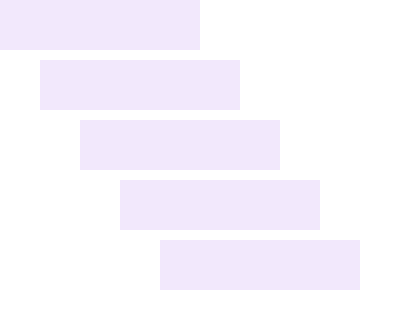## Challenge 2

Create a for loop that will run through the loop ten times. Each loop draws a rectangle positioned at x = 10 with a height of 20. The first rectangle is positioned at (10, 10) with a width of 80. Each rectangle is 30 pixels wider than the previous rectangle, and each rectangle is drawn below the previous rectangle with a space that is 5-pixels tall between them. Fill all of the rectangles with the color `'MediumSeaGreen'`.

Message Log
##### Canvas (your drawing will display here)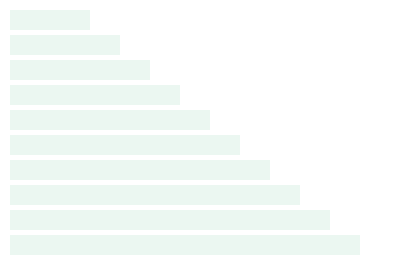## Use Transformations in a For Loop

While using the value of the counter to position drawings inside of a for loop works, it's often simpler to position drawings by using transformations. You will need to be familiar with the `context.save()` and `context.translate()` methods to understand this example and complete the following challenge.

In this example, we draw a grid of lines using two separate for loops. We start by translating to the top left corner of the grid, which is at coordinates (0, 0), setting the `context.strokeStyle` property, and saving the drawing state:

``````context.translate(10, 10);
context.strokeStyle = 'Crimson';
context.save(); // The origin of the coordinate system is at the top left corner of the grid``````

We save the drawing state because we will want to restore the origin of the coordinate system back to the top left corner of the grid later.

Next, we create the first for loop to draw 17 vertical lines that are 240 pixels long and 20 pixels apart.

``````for (var i = 0; i < 17; i = i + 1) {
context.beginPath();
context.moveTo(0, 0);
context.lineTo(0, 240);
context.stroke();
context.translate(20, 0);
}``````

Note that we are drawing each line from (0, 0) to (0, 240). To position each line 20 pixels over from the previous line, we use the `context.translate()` method to move the entire coordinate system 20 pixels to the right.

Once the first for loop is finished, we are ready to draw the horizontal lines in our grid, but the origin of the coordinate system is currently 20 pixels to the right of the last vertical line drawn. Before we can draw our horizontal lines, we need to restore the origin back to the top left corner of the grid:

``context.restore(); // Restores the origin of the coordinate system back to the top left corner of the grid``

Finally, we create the second for loop to draw 13 horizontal lines that are 320 pixels long and 20 pixels apart.

``````for (var j = 0; j < 13; j = j + 1) {
context.beginPath();
context.moveTo(0, 0);
context.lineTo(320, 0);
context.stroke();
context.translate(0, 20);
}``````

Once again, we are drawing the same line from (0, 0) to (320, 0) each time through the loop. To position each line 20 pixels down from the previous line, we use the `context.translate()` method to move the entire coordinate system 20 pixels down.

Because the second for loop happens after the first for loop, we could have used the same counter, `i`, for both loops. If we re-used the variable `i` in the second loop, we would still initialize it by assigning it the value of 0, but we would not declare it again.

To learn more about saving and restoring the drawing state, translating the coordinate system, and drawing lines, visit the and lineTo() lessons.

Quick Reference:

Message Log

## Challenge 3

Create a for loop that will run through the loop twelve times. Each loop draws a square with a width and height of 20. The first square is positioned at (100, 20), and the top left corner of the next square touches the bottom right corner of the previous square. Fill all of the squares with the color `'SaddleBrown'`. Use the `context.translate()` method to position the squares.

Message Log
##### Canvas (your drawing will display here)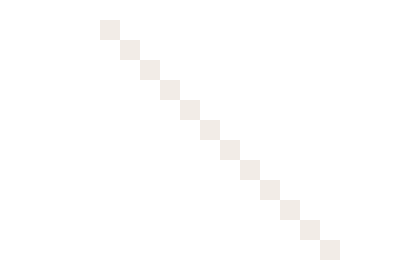## Shortcuts for Incrementing the Loop

So far, we have been using the statement `i = i + 1` to increment our counter by 1 each time through the loop. Because incrementing variables is so common in programming, there are a number of shortcuts for writing the same statement.

Writing `i += 10` is a shorter way to write `i = i + 10` and `i -= 5` is a shorter way to write `i = i - 5`. We can even write `i++` for `i = i + 1` and `i--` for `i = i - 1`. While shortcuts mean less typing, code should also be easy to read and understand.

In this example, we change how we implement the counters in our for loops and make our program more flexible.

We start by declaring three variables:

``````var gridWidth = 320;
var gridHeight = 240;
var gridSpace = 20;``````

Next, we update the condition and increment statement in the first for loop:

``````for (var i = 0; i <= gridWidth; i += gridSpace) {
context.beginPath();
context.moveTo(0, 0);
context.lineTo(0, gridHeight);
context.stroke();
context.translate(gridSpace, 0);
}``````

Instead of hardcoding the number of vertical lines drawn by the for loop, we simply intialize the counter `i = 0` and increment it by the amount of space between grid lines. This means the value of `i` is the x-coordinate of the next line. We continue drawing lines as long as `i <= gridWidth`.

Inside of the for loop, we draw the vertical grid lines so they are as long as the height of the grid, and we shift the coordinate system to the right based on the amount of space between grid lines.

Finally, we make similar changes to the second for loop:

``````for (var j = 0; j <= gridHeight; j += gridSpace) {
context.beginPath();
context.moveTo(0, 0);
context.lineTo(gridWidth, 0);
context.stroke();
context.translate(0, gridSpace);
}``````

Now we can adjust the size and spacing in the grid simply by assigning new values to the `gridWidth`, `gridHeight`, and `gridSpace` variables, and we will have a nice grid as long as the `gridWidth` and `gridHeight` are evenly divisible by the `gridSpace`.

Quick Reference:

Message Log

## Challenge 4

Create a for loop that assigns the counter `i` an intial value of 0, increments `i` by 15 each time through the loop, and continues running as long as the value of `i` is less than or equal to 255.

Inside the for loop's code block, use the counter `i` to set the `context.fillStyle` property to a shade of blue:

``context.fillStyle = 'rgb(0, 0, ' + i + ')';``

Then, draw a filled rectangle with a width of 20 and height of 200. The first rectangle is positioned at (10, 10), and the next rectangle is positioned just to the right of the previous one. Use the `context.translate()` method to position the rectangles.

Message Log
##### Canvas (your drawing will display here)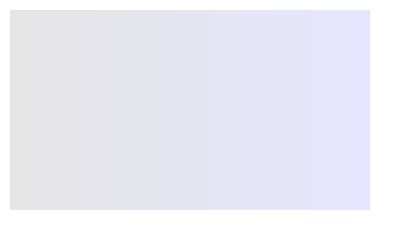## Iterate Over an Array

For loops are often used to iterate over arrays, which are lists of values.

In this example, we start by declaring a variable named `colors` and assign it an array of HTML5 color names:

``var colors = ['Red', 'Orange', 'Yellow', 'Green', 'Blue', 'Indigo', 'Violet'];``

To iterate over the array `colors` using a for loop, we assign the counter an intial value of 0, increment the counter by 1, and use `i < colors.length` as the condition:

``````for (var i = 0; i < colors.length; i += 1) {

// code block

}``````

Because there are seven elements in the array, `colors.length` is 7. By using `i < colors.length` as our condition, we will run through the loop seven times, once per element.

Inside the for loop's code block, we set the `context.fillStyle` property to `colors[i]`. In the first loop, `i == 0`, and `colors` accesses the first element in the array, which is the color `'Red'`. In the second loop, `i == 1`, and `colors` accesses the second element in the array, which is the color `'Orange'`. The elements in an array are indexed starting at the number zero.

``````for (var i = 0; i < colors.length; i += 1) {

context.fillStyle = colors[i];

}``````

Finally, we draw a rectangle with the fill color and translate the origin of the coordinate system to draw the rectangle in the next loop.

``````for (var i = 0; i < colors.length; i += 1) {
context.fillStyle = colors[i];
context.fillRect(0, 0, 40, 240);
context.translate(40, 0);
}``````

You can change the drawing simply by changing the colors listed in the array. Here is a full list of If you add or remove colors, the for loop will run through the correct number of loops because it is using `i < colors.length` as its condition, and the value of the `colors.length` property is automatically updated.

To learn more about arrays and fill colors, visit the Arrays and fillStyle lessons.

Quick Reference:

Message Log

## Challenge 5

Create a for loop to iterate over the array `data` provided below. The for loop will use the list of values stored in the array `data` to draw a bar graph.

The top left corner of the bar graph is at (10, 10). Each bar is positioned at x = 10 with a height of 20. The width of the first bar is the value accessed by `data`, the width of the second bar is the value accessed by `data`, etc. The vertical space between bars is 5-pixels tall, and all of the bars are filled with the color `'MediumSeaGreen'`.

Message Log
##### Canvas (your drawing will display here)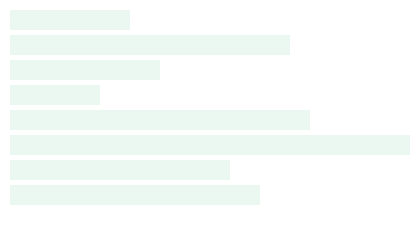## Nested For Loops

To draw a grid of squares, we are going to use a for loop inside of another for loop. The inner for loop will draw a row of squares. The outer for loop will draw a column of rows.

The number of rows in our grid will be determined by the number of colors in the array `colors.` At the moment, there are seven colors in the array, so there will be seven rows. All of the squares in a row will have the same color.

We start by setting up the outer for loop first:

``````var colors = ['Red', 'Orange', 'Yellow', 'Green', 'Blue', 'Indigo', 'Violet'];

for (var i = 0; i < colors.length; i += 1) {
context.save(); // Save the drawing state with the origin at the start of the row
context.fillStyle = colors[i];

// Draw row of squares

context.restore(); // Restore the origin back to the start of the row
context.translate(0, 40); // Move the origin down 40 pixels to the start of the next row
}``````

The first thing we do in the code block for the outer for loop is save the drawing state. When drawing a row of squares, we use the `context.translate()` method to move the origin of the coordinate system down the row. We need some way to return to the start of the row so we can be ready to draw the next row. That's why we call the `context.restore()` method after drawing the row of squares. Then, we use the `context.translate()` method to move the origin down to the start of the next row. Before drawing the row of squares, we set the `context.fillStyle` property to the color accessed from the array at index `i`.

With the outer for loop set up, the inner for loop is fairly simple. We run through the inner loop ten times, drawing a square filled with the color stored in the `context.fillStyle` property and then moving the origin of the coordinate system over each time.

``````var colors = ['Red', 'Orange', 'Yellow', 'Green', 'Blue', 'Indigo', 'Violet'];

for (var i = 0; i < colors.length; i += 1) {
context.save(); // Save the drawing state with the origin at the start of the row
context.fillStyle = colors[i];

for (var j = 0; j < 10; j += 1) {
context.fillRect(0, 0, 30, 30);
context.translate(40, 0); // Move the origin over 40 pixels to draw the next square
}

context.restore(); // Restore the origin back to the start of the row
context.translate(0, 40); // Move the origin down 40 pixels to the start of the next row
}``````

Because the inner for loop is inside of the outer for loop, we have to use different counters for both loops. Also, indenting the code blocks makes the code much easier to read once there are code blocks inside of other code blocks.

Quick Reference:

Message Log

## Challenge 6

Use nested for loops to create a grid of rectangles filled with the color `'SlateBlue'`.

Each rectangle has a width of 30 and a height of 20. There are ten rectangles in a row and eight rows in the column. The rectangle in the top left corner is positioned at (10, 10). The spacing between rows, both horizontally and vertically, is 10 pixels.

Message Log
##### Canvas (your drawing will display here)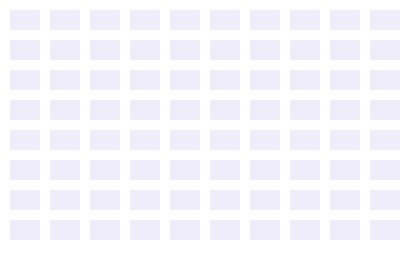## Break out of a For Loop

There are times when we want to break out of a for loop before its condition returns false.

In this example, we use a for loop to iterate over the array `colors`. Just like we did before, we draw a rectangle with each color in the array, except this time we break out of the loop, ending it early, if we find the color `'Blue'`.

``````var colors = ['Red', 'Orange', 'Yellow', 'Green', 'Blue', 'Indigo', 'Violet'];

context.translate(20, 20);

for (var i = 0; i < colors.length; i += 1) {
if (colors[i] == 'Blue') {
break;
}

context.fillStyle = colors[i];
context.fillRect(0, 0, 40, 240);
context.translate(40, 0);
}``````

Inside of the for loop, we have added an if statement to check if `colors[i] == 'Blue'`. If the condition inside the if statement returns true, then we use a break statement to break out of the loop. Note that our for loop only draws the first four rectangles even though there are seven colors in the array.

To learn more about if statements, visit the If Statements lesson.

Quick Reference:

Message Log

## Use a For Loop to Delete Elements in an Array

There are also times when we want to use a for loop to iterate over an array in order to find elements that meet a certain condition and delete them.

In this example, we use a for loop to iterate over the array `colors`. If we find the color `'Yellow'` in the array, we delete the color from the array. While this process is fairly straightforward, there is one complication. If we start from the beginning of the array, find the color `'Yellow'` in the array at index 2, and delete it—the index of the color `'Green'` suddenly becomes 2 and the index of the color `'Blue'` suddenly becomes 3.

Then, when the for loop repeats and goes to check the color at index 3, it skips the color `'Green'` entirely and finds the color `'Blue'` instead. If the color `'Yellow'` only appears in the array once, this is not a problem. But it is a problem if the color `'Yellow'` is in the array at two consecutive indices or if we are doing more than deleting elements from the array.

The best way to loop through an array and delete elements is to start at the end of the array and work backwards.

``````var colors = ['Red', 'Orange', 'Yellow', 'Green', 'Blue', 'Indigo', 'Violet'];

for (var i = colors.length - 1; i >= 0; i -= 1) {
if (colors[i] == 'Yellow') {
colors.splice(i, 1);
} else {
context.fillStyle = colors[i];
context.fillRect(0, 0, 40, 240);
context.translate(40, 0);
}
}``````

We initialize the counter using `var i = colors.length - 1` because `colors.length - 1` is the index of the last element. Remember, the index of an array starts at zero. We decrement the counter by subtracting 1 from it at the end of each loop until the condition `i >= 0` returns false.

Inside of the for loop, we use an if statement to check if the color is `'Yellow'`. If the condition inside of the if statement returns true, we use the array's `splice()` method to delete the element at index `i`. But if the statement returns false, we draw a rectangle filled with the color.

Note that the colors in the array are drawn in reverse order and the color `'Yellow'` is not included. We have successfully deleted it from the array.